Refer to our Texas Go Math Grade 3 Answer Key Pdf to score good marks in the exams. Test yourself by practicing the problems from Texas Go Math Grade 3 Lesson 4.3 Answer Key Use Properties to Add.

Unlock the Problem

Mrs. Gomez sold 36 cucumbers, 37 tomatoes, and 51 peppers at the Farmers’ Market. How many vegetables did she sell?
Will the sum be closer to 120 or 150?
closer to 120
Explanation:
Mrs. Gomez sold 36 cucumbers, 37 tomatoes, and 51 peppers at the Farmers’ Market.
Total vegetables she sold
36 + 37 + 51 =124
the sum is closer to 120

Use properties to find 36 + 37 + 51.
STEP 1:
Line up the place values.
36 + 37 + 51 =124.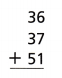STEP 2:
Change the grouping.

Think: Adding 37 + 51 first would be easy because there is no regrouping needed.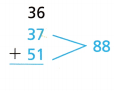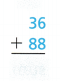So, Mrs. Gomez sold ___________.
Explanation:
Line up the place values, and Change the grouping.
So, no regrouping is required.

Try This!

Subtraction is not commutative or associative. Perform operations in parentheses first.

Then subtract from left to right.
A.
(88 – 34) – 10 = _________
54 – 10 = 44
B.
88 – (34 – 10) = __________
88 – 44 = 44

Explain how you can use your answers to show that subtraction is not associative.
Subtraction is not an associative operation.
This is because it does not satisfy the associative property.
For example,
The answer remain same as 44 in the below equation.
88 – 44 or 54 – 10
Explanation:
The associative property in math is the property of numbers that states the sum or the product of three or more numbers will not change in whatever sequence numbers are grouped.
Subtraction is not an associative operation.
This is because it does not satisfy the associative property.

Share and Show

Question 1.
Find the sum. Write the addition property you used.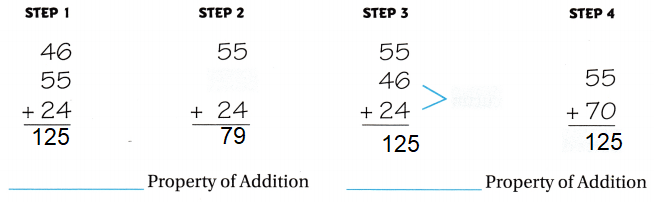Explanation:
The associative property in math is the property of numbers that states the sum or the product of three or more numbers will not change in whatever sequence numbers are grouped.

Use addition properties and strategies to find the sum.

13 + 26 + 54 = _________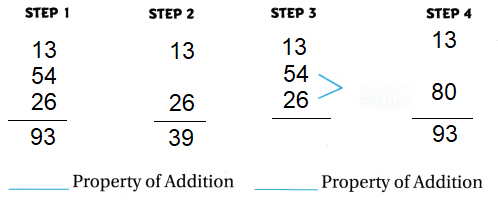Explanation:
In Commutative property, when two numbers are added, the sum is the same regardless of the order of the addends.
Commutative Property x + y = y+ x
Associative property states that the sum of three or more numbers will not change in whatever sequence numbers are grouped.
Associative Property x + (y + z) = (x + y) + z
13 + (54 + 26) = 93 =(13 + 54)+ 26

Question 3.
57 + 62 + 56 +43 = _________
Explanation:
As said in the above one can use Associative or Commutative properties.
(57 + 43) + (62 + 56) = 100 + 118 = 218

Math Talk
Mathematical Processes

Explain how the Commutative and Associative Properties of Addition are alike and how they are different.
The Commutative and Associative Properties of Addition are alike:
Both commutative and associative properties are rules applied to addition and multiplication operations.
These properties are laws used in algebra to help solve problems.
The difference between Commutative and Associative Properties of Addition are:
The commutative property comes from the term “commute” which means ‘move around’ and it refers to being able to switch numbers that you’re adding or multiplying regardless of the order of the numbers.
The associative property, on the other hand, comes from the word “associate” or “group” and it refers to grouping of three or more numbers using parentheses, regardless of how you group them. The result will be the same, no matter how you re-group the numbers or variables.

Question 4.
Change the order and the grouping of the addends so that you can use mental math to find the sum. Then find the sum.
43 + 39 + 43 + 11 = _________
_________ + _________ + _________ + _________= _________
Explanation:
By breaking up numbers into separate parts and than adding up the matching parts,
and than adding up the sums to find the difference.
(43 + 39) + (43 + 11) = 82 + 54 = 136

Problem Solving

Question 5.
Apply Mr. Arnez bought 32 potatoes, write Math show Your Work 29 onions, 31 tomatoes and 28 peppers to make salads for his deli. How many vegetables did he buy?
Explanation:
Mr. Arnez bought 32 potatoes, 29 onions, 31 tomatoes and 28 peppers to make salads for his deli.
Total vegetables he bought
32 + 29 +28 = (32 +28) + 29 = 60 + 29 = 89

Multi-Step Ms. Chang is baking pies and cakes for the school bake sale. She bought 16 apples, 29 peaches, and 11 bananas at the Farmers’ Market. How many pieces of fruit did she buy?
Explanation:
Ms. Chang bought 16 apples, 29 peaches, and 11 bananas at the Farmers’ Market.
Total pieces of fruit she bought
16 + 29 + 11 = 56

Question 7.
Multi-Step Mr. Staples bought 48 paper plates, 22 paper cups, and 50 paper napkins for the picnic. How many paper products did he buy?
Explanation:
Mr. Staples bought 48 paper plates, 22 paper cups, and 50 paper napkins for the picnic.
Total paper products he bought
48 + 22 + 50 = 70 + 50 = 120

Question 8.
H.O.T. Communicate What is the unknown number? Which property did you use?
(☐ + 8) + 32 = 49
Explanation:
Associative Property x + (y + z) = (x + y) + z
let n be a unknown number
(n+8) + 32 = 49
(n+8) = 49 – 32
n + 8 = 17
n = 9

Question 9.
H.O.T. Multi-Step Change the order or grouping to find the sum. Explain how you used properties to find the sum.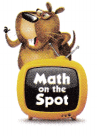63 + 86 + 77
Explanation:
Associative property can be used to find the sum
Associative Property x + (y + z) = (x + y) + z
(63 + 77) + 86 = 140 + 86 = 226
63 + (77 + 86) = 63 + 163 = 226

Fill in the bubble for the correct answer choice. Use properties to solve.

Question 10.
Josephine took photos of three canyons during the rafting trip. In the first canyon, she took 64 photos. She took 31 photos of the second canyon. In the third canyon, she took 49 photos. How many photos did Josephine take in all?
(A) 154
(B) 144
(C) 124
(D) 164
Option (B)
Explanation:
In the first canyon, she took 64 photos.
She took 31 photos of the second canyon.
In the third canyon, she took 49 photos.
Total photos did Josephine took in all
64 + (31 + 49) = 64 + 80 = 144

Tim got the answer 80 to the problem (97 – 22) – 5. Which statement shows the error he made?
(A) He regrouped the numbers as 97 – (22 – 5).
(B) He subtracted the numbers in parentheses first.
(C) He did not subtract all the numbers.
(D) He subtracted from left to right.
Option (B)
Explanation:
Because he subtracted the numbers in parentheses first.
97 – (22 – 5)
97 – 17 = 80

Question 12.
Multi-Step A travel company offers rafting trips for families. The fees for the trip are $54 for adults and$46 for children. The Kirk family of two adults and two children want to go on the trip. How much will they pay for their rafting trip?
(A) $200 (B)$300
(C) $220 (D)$320
Option (A)
Explanation:
The fees for the trip are $54 for adults and$46 for children.
The Kirk family of two adults = 54 X 2 = 108
two children fees = 46 X 2 = 92
Total amount they paid for their rafting trip
(54 + 46) + (54 + 46) = 100 + 100 = 200

Texas Test Prep

Communicate Which shows the Associative Property of Addition?
(A) (86 + 7) + 93 = 86 + (7 + 93)
(B) 86 + 7 = 7 + 86
(C) 86 + 0 = 86
(D) 86 = 80 + 6
Explanation:
Both commutative and associative properties are rules applied to addition operations.
(86 + 7) + 93 = 86 + (7 + 93)

### Texas Go Math Grade 3 Lesson 4.3 Homework and Practice Answer Key

Find the sum. Write the addition property you used.

Question 1.
28 + 29 + 42 = __________
Explanation:
Associative Property x + (y + z) = (x + y) + z
(28 + 29 )+ 42 = 57 + 42 = 99
(28 + 42) + 29 = 70 + 29 = 99

Question 2.
23 + 36 + 77 = __________
Explanation:
Associative Property x + (y + z) = (x + y) + z
(23 + 77) + 36 = 23 + (77 + 36)
100 + 36 = 23 + 113
136 = 136

Question 3.
25 + 48 + 125 = __________
Explanation:
Associative Property x + (y + z) = (x + y) + z
(25 + 48) + 125 = 25 + (48 + 125)
73 + 125 = 25 + 173
198 = 198

Question 4.
34 + 22 + 46 + 48 = __________
Explanation:
Associative Property x + (y + z) = (x + y) + z
34 + 22 + 46 + 48
56 + 46 + 48
(56 + 46) + 48 = 56 + (46 + 48 )
102 + 48 = 56 + 94
150 = 150

21 + 55 + 39 + 45 = __________
Associative Property x + (y + z) = (x + y) + z
Explanation:
21 + 55 + 39 + 45
(21 + 55) + 39 + 45
76 + 39 + 45
(76 + 39) + 45 =76 + (39 + 45)
115 + 45 = 76 + 84
160 = 160

Question 6.
62 + 27 + 48 = __________.
Associative Property x + (y + z) = (x + y) + z
Explanation:
(62 + 27) + 48 = 62 + (27 + 48)
89 + 48 = 62 + 75
137 = 137

Problem Solving

Question 7.
The Smoothie Stop sold 35 banana smoothies, 48 strawberry smoothies, and 25 orange smoothies. How many smoothies were sold in all?
108 smoothies
Explanation:
The Smoothie Stop sold 35 banana smoothies, 48 strawberry smoothies, and 25 orange smoothies.
Total smoothies sold in all
35 + 48 + 25 =
(35 + 25) + 48
60 + 48 =108

Question 8.
Max spent 28 minutes on math homework, 27 minutes on science homework, and 22 minutes on English homework. How much time did he spend on homework?
78 MINUTES
Explanation:
Max spent 28 minutes on math homework, 27 minutes on science homework, and 22 minutes on English homework.
Total time he spent on homework
28 +28 + 22
(28 + 22)+ 28
50 +28 = 78

Lesson Check

Question 9.
Students collected used books to sell at the school fair. On Wednesday, 74 books were donated. On Thursday, 62 books were collected. On Friday, students brought in 36 books. How many books were collected on all three days?
(A) 162
(B) 182
(C) 172
(D) 170
Option (C)
Explanation:
On Wednesday, 74 books were donated.
On Thursday, 62 books were collected.
On Friday, students brought in 36 books.
Total books collected on all three days
74 + 62 +36 = 172

Local farmers had a booth at the school fair. Farmers sold 53 pounds of squash, 58 pounds of tomatoes, and 37 pounds of zucchini. How many pounds of vegetables were sold in all?
(A) 148 pounds
(B) 90 pounds
(C) 158 pounds
(D) 138 pounds
Option (A)
Explanation:
Farmers sold 53 pounds of squash,
58 pounds of tomatoes,
and 37 pounds of zucchini.
Total pounds of vegetables sold in all
53 + 58 + 37 =
(53 + 37) + 58 = 90 + 58 = 148

Question 11.
Mrs. Jackson is in charge of the food booth. She made a supply list. How many packages of paper goods are on the supply list?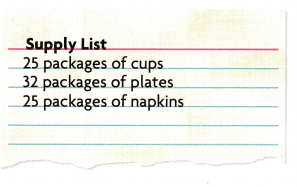(A) 92
(B) 82
(C) 72
(D) 50
Option (B)
Explanation:
In the given supply list there are
25 packages of cups
32 packages of plates
25 packages of napkins
Total packages all together
25 + 32 + 25 = 82

Multi-Step There was a food drive at the school fair. Two local grocery stores each donated the number of food packages shown in the table. How many packages of food did both stores donate altogether?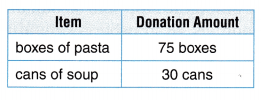(A) 105
(B) 135
(C) 210
(D) 150